Picture by Robert Bye, Unsplash.

Linear regression models are widely used in every industry. They predict a number from a range of other features based on a linear relationship between the input variables (X) and the output variable (y).

Linear models are quick and easy to build and can work very well when properly tuned. You can use them for almost anything, from predicting the value of stocks and shares, to forecasting tomorrow’s weather.

In this project we’re going to build a really simple linear regression model to predict the value of housing in California. At the end, you should be able to apply the same techniques for creating linear models to examine the data of your choice.

Open a new Jupyter notebook and enter the lines below to load the packages we’ll be using for this simple project. If you don’t have the packages installed already, you can install them from within your Jupyter notebook by entering `!pip3 install pandas` (where `pandas` is the name of the missing package) and then executing the code cell by holding shift and pressing enter.

``````import pandas as pd
import numpy as np
import seaborn as sns
import matplotlib.pyplot as plt
from pandas.plotting import scatter_matrix
from sklearn.preprocessing import LabelEncoder
from sklearn.preprocessing import StandardScaler
from sklearn.model_selection import train_test_split
from sklearn.linear_model import LinearRegression
from sklearn.metrics import mean_squared_error
``````

The data set we’re using is a Kaggle data set which includes details on house prices in different parts of California. We’ll be using the features in here (such as the latitude and longitude, number of rooms, and the proximity to the ocean) to predict the `median_house_value` using a linear regression model.

We’ll use the Pandas `read_csv()` function to load our data set into a dataframe called `df`, and then print the first five lines of the file using `df.head()`. I’ve used the `.T` suffix to transpose the data so it fits better on the page.

``````df = pd.read_csv('https://raw.githubusercontent.com/flyandlure/datasets/master/housing.csv')
``````
0 1 2 3 4
longitude -122.23 -122.22 -122.24 -122.25 -122.25
latitude 37.88 37.86 37.85 37.85 37.85
housing_median_age 41 21 52 52 52
total_rooms 880 7099 1467 1274 1627
total_bedrooms 129 1106 190 235 280
population 322 2401 496 558 565
households 126 1138 177 219 259
median_income 8.3252 8.3014 7.2574 5.6431 3.8462
median_house_value 452600 358500 352100 341300 342200
ocean_proximity NEAR BAY NEAR BAY NEAR BAY NEAR BAY NEAR BAY

### Check the data types

Our model needs to have numeric data from which to make its predictions, so we’ll use the `df.info()` command to examine the data types by column. Everything looks fine, as we mostly have `float64` numeric data, apart from in the `ocean_proximity` column which contains a text string Pandas classifies as an `object` data type. This is known as a categorical variable.

``````df.info()
``````
``````<class 'pandas.core.frame.DataFrame'>
RangeIndex: 20640 entries, 0 to 20639
Data columns (total 10 columns):
#   Column              Non-Null Count  Dtype
---  ------              --------------  -----
0   longitude           20640 non-null  float64
1   latitude            20640 non-null  float64
2   housing_median_age  20640 non-null  float64
3   total_rooms         20640 non-null  float64
4   total_bedrooms      20433 non-null  float64
5   population          20640 non-null  float64
6   households          20640 non-null  float64
7   median_income       20640 non-null  float64
8   median_house_value  20640 non-null  float64
9   ocean_proximity     20640 non-null  object
dtypes: float64(9), object(1)
memory usage: 1.6+ MB
``````

### Encode the categorical variable

The categorical variable `ocean_proximity` needs to be turned into a numeric value so it can be used by our model. There are several ways we could “encode” the data within, but choosing the right solution depends on the underlying data. Specifically, we need to understand the “cardinality” of the column and identify how many unique values it holds, which we can do by appending `.value_counts()` to the `ocean_proximity` column of our dataframe.

``````df['ocean_proximity'].value_counts()
``````
``````<1H OCEAN     9136
INLAND        6551
NEAR OCEAN    2658
NEAR BAY      2290
ISLAND           5
Name: ocean_proximity, dtype: int64
``````

The `value_counts()` function reveals that we have quite low cardinality, with just five unique values across this column. This is well suited to a categorical variable encoding technique called one-hot encoding. One-hot encoding basically “binarises” the data and adds new columns with a value of 1 or 0, depending on whether a row matches. This will give us five new columns on our dataframe, with four set to 0 and one set to 1.

The one-hot encoding step is handled for us by a Pandas function called `get_dummies()`. This takes the column values (i.e. NEAR OCEAN) and uses them in the column name. That often doesn’t look very neat, so I prefer to “slugify” the column values first by converting them to lowercase letters and stripping out any other characters.

``````df['ocean_proximity'] = df['ocean_proximity'].str.lower().replace('[^0-9a-zA-Z]+','_',regex=True)
``````

Now the column values have been tidied up, we will use `get_dummies()` to one-hot encode the data and prefix all the new columns with `proximity` so they’re easier to identify. The one-hot encodings get assigned to a dataframe called `encodings` which we will then concatenate (or join) to the side of the `df` dataframe. If we then print a sample of five random rows we’ll see the new columns.

``````encodings = pd.get_dummies(df['ocean_proximity'], prefix='proximity')
df = pd.concat([df, encodings], axis=1)
df.sample(5).T
``````
10548 12365 6490 7303 15707
longitude -117.77 -116.47 -118.01 -118.19 -122.43
latitude 33.7 33.81 34.1 33.98 37.79
housing_median_age 3 7 35 40 50
total_rooms 3636 10105 2120 973 3312
total_bedrooms 749 2481 412 272 1095
population 1486 6274 1375 1257 1475
households 696 2095 405 258 997
median_income 5.5464 2.4497 3.4609 2.8214 2.7165
median_house_value 207500 90900 166300 158000 500001
ocean_proximity _1h_ocean inland inland _1h_ocean near_bay
proximity__1h_ocean 1 0 0 1 0
proximity_inland 0 1 1 0 0
proximity_island 0 0 0 0 0
proximity_near_bay 0 0 0 0 1
proximity_near_ocean 0 0 0 0 0

### Exploratory Data Analysis

The next step is called Exploratory Data Analysis or EDA. This is designed to help you understand the data to see what further work may be required to transform it for use by the model. The basics are quite straightforward, but in-depth EDA does require detailed knowledge of statistics and the ways in which models work, so we’ll sidestep this a bit for simplicity and just cover the basic principles instead.

#### Visualise the data with histograms

One of the most useful first steps with any new data set is to examine the spread of the data (or the statistical distribution) by using Pandas to plot histograms. Histograms are basically bar charts which automatically “bin” the data. This removes a bit of the detail (depending on the number of bins you use) and can be a good way to get an overview of where the data lie. The two lines of code below handle all of this for us.

``````df.hist(bins=50, figsize=(20,15))
plt.show()
``````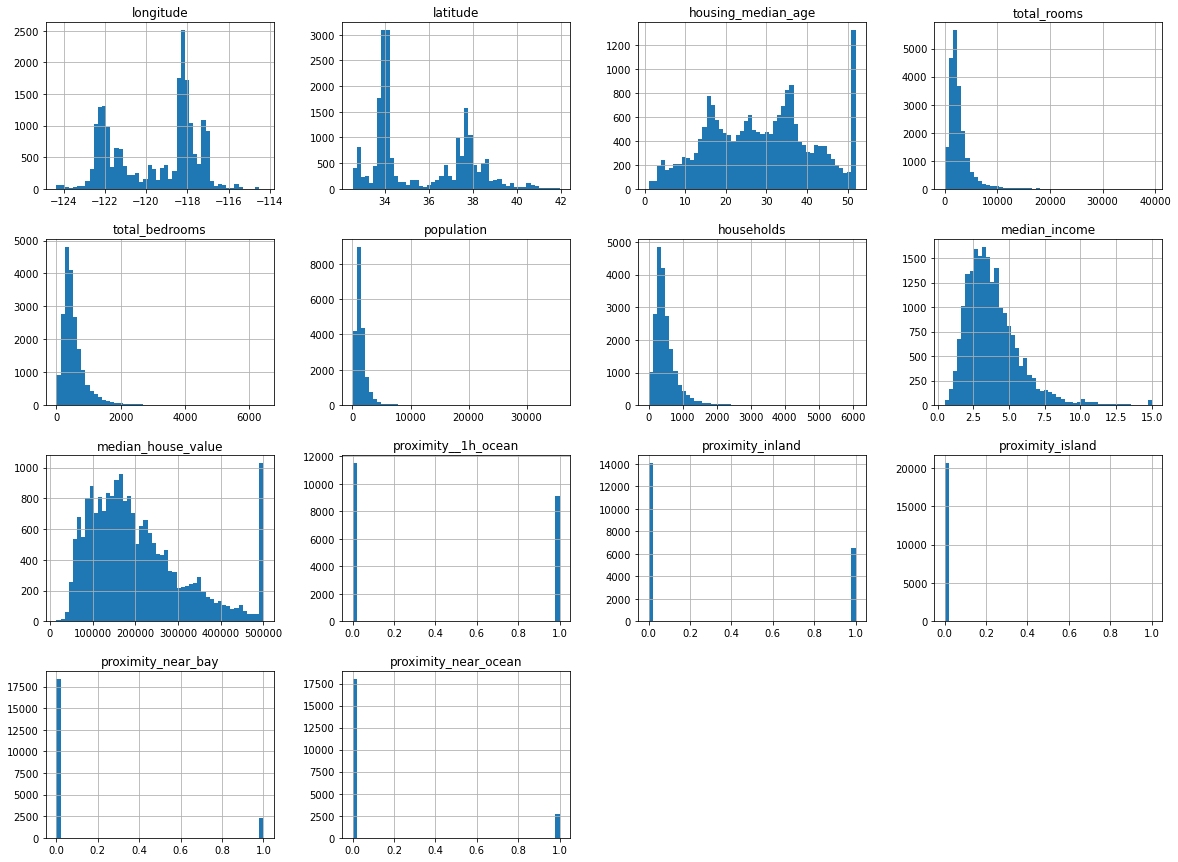The histograms for the proximity data we one-hot encoded show that it’s binary, as there are only values at either end. The other data are distributed either with a left skew (i.e. mostly low values), or a more “normal” distribution where the values are spread out a bit more evenly.

Any big spikes that sit away from other points could represent outliers, or statistical anomalies. These can often throw a model off track. If you look at the `housing_median_age` histogram you can see a very clear spike around the 50 mark, which represents a clear outlier. We’ll skip the removal of this for now for simplicity, but doing so will give us an extra performance boost later on.

#### Examine the summary statistics

The summary statistics for the data cover its count, mean, standard deviation, minimum value, maximum value and the inter-quartile range. Ordinarily, by examining the values in here, you might choose to create other visualisations of individual columns to help you understand the data in more detail, but we’ll overlook this for now.

``````df.describe().T
``````
count mean std min 25% 50% 75% max
longitude 20640.0 -119.569704 2.003532 -124.3500 -121.8000 -118.4900 -118.01000 -114.3100
latitude 20640.0 35.631861 2.135952 32.5400 33.9300 34.2600 37.71000 41.9500
housing_median_age 20640.0 28.639486 12.585558 1.0000 18.0000 29.0000 37.00000 52.0000
total_rooms 20640.0 2635.763081 2181.615252 2.0000 1447.7500 2127.0000 3148.00000 39320.0000
total_bedrooms 20433.0 537.870553 421.385070 1.0000 296.0000 435.0000 647.00000 6445.0000
population 20640.0 1425.476744 1132.462122 3.0000 787.0000 1166.0000 1725.00000 35682.0000
households 20640.0 499.539680 382.329753 1.0000 280.0000 409.0000 605.00000 6082.0000
median_income 20640.0 3.870671 1.899822 0.4999 2.5634 3.5348 4.74325 15.0001
median_house_value 20640.0 206855.816909 115395.615874 14999.0000 119600.0000 179700.0000 264725.00000 500001.0000
proximity__1h_ocean 20640.0 0.442636 0.496710 0.0000 0.0000 0.0000 1.00000 1.0000
proximity_inland 20640.0 0.317393 0.465473 0.0000 0.0000 0.0000 1.00000 1.0000
proximity_island 20640.0 0.000242 0.015563 0.0000 0.0000 0.0000 0.00000 1.0000
proximity_near_bay 20640.0 0.110950 0.314077 0.0000 0.0000 0.0000 0.00000 1.0000
proximity_near_ocean 20640.0 0.128779 0.334963 0.0000 0.0000 0.0000 0.00000 1.0000

#### Examine correlations with the target

Our linear regression model is going to predict the `median_house_value` based on the other features. In order to be able to do this, there needs to be some kind of mathematical relationship - or “correlation” - between the features and the target.

A clever statistical calculation called the Pearson product moment correlation is usually used to calculate this. It returns a “correlation coefficient”. If this is close to 1 then there’s a perfect correlation, if it’s close to 0 then there’s little or no correlation, while if it’s less than 1 there’s a negative correlation.

The three lines below create a heatmap correlation matrix showing these correlation coefficients for each pair of variables. You can see from the below that there’s a strong link between `median_income` and `median_house_value`, and that `proximity__1h_ocean` (properties within an hour of the ocean) are associated with a higher value. There should be some useful data here for our model to use.

``````plt.figure(figsize=(14,8))
corr = df.corr()
heatmap = sns.heatmap(corr, annot=True, cmap="Blues")
``````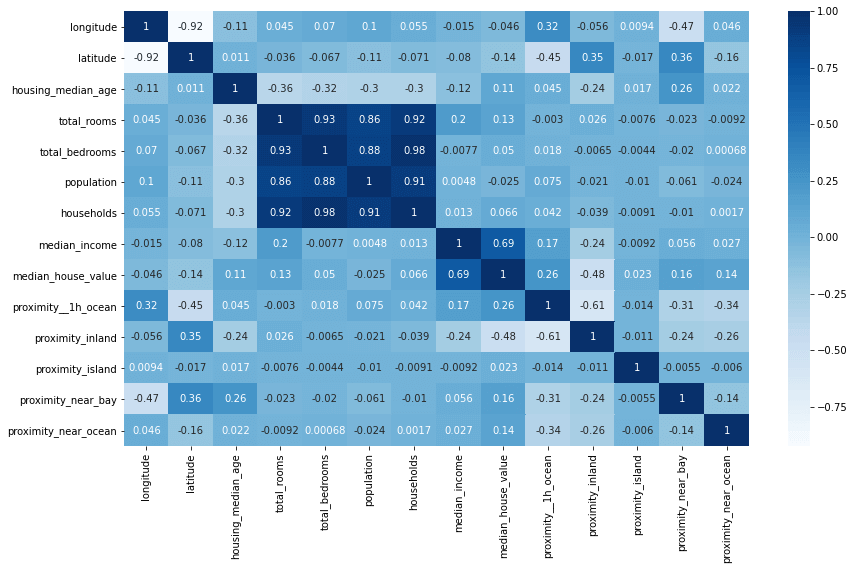It can also be useful to plot the correlations on a bar chart. This shows the correlation coefficient for each column against the target column we’re trying to predict. There’s a clear positive link between income, proximity to the ocean, the Bay area, the number of rooms and the median age.

``````plt.figure(figsize=(14,8))
bars = df.corr()['median_house_value'].sort_values(ascending=False).plot(kind='bar')
``````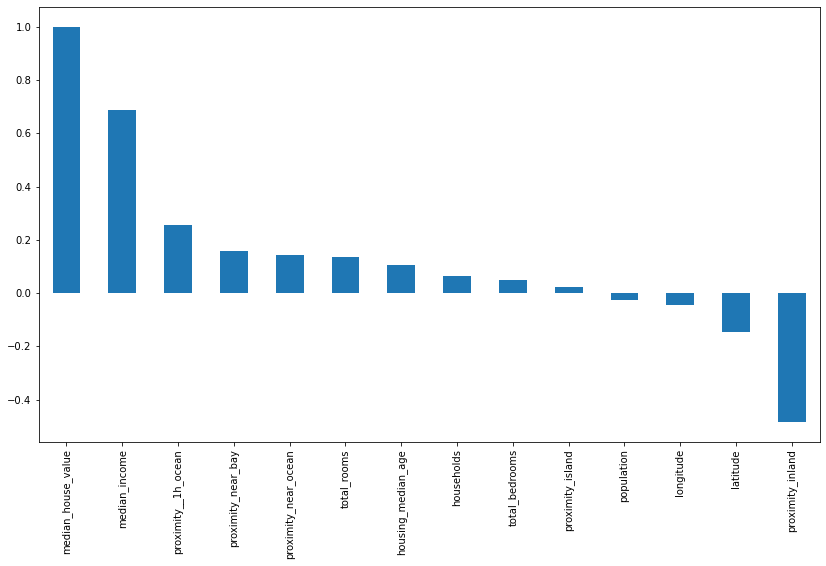#### Location, location, location

To check whether the location of the property makes a difference we can create a scatterplot of the `latitude` and `longitude` values which effectively creates a crude map of the locations of the houses. You can see that some areas have a higher density of values, denoting a higher population, but the really interesting bit is in the colour of the dots. By using a colour map (or `cmap`) set to the `median_house_value` column, we can see that it’s the houses along parts of the California coastline which have the highest median values.

``````df.plot(
x='longitude', y='latitude',
kind='scatter', figsize=(10,7),
alpha=.4,
c='median_house_value', cmap=plt.get_cmap('jet'), colorbar=True
)
``````
``````<matplotlib.axes._subplots.AxesSubplot at 0x7f52780e0160>
``````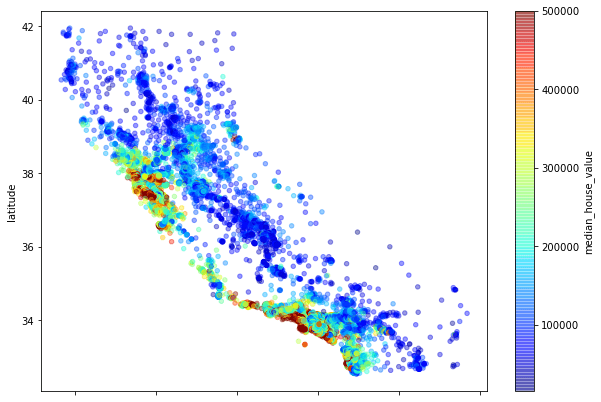#### Examine missing values

The other important step during EDA is to identify whether there are any missing values in the dataset. By default, these will be present as a `NaN` or null value and the model won’t be able to handle these. There are two main options when it comes to missing values, you can either drop them or “impute” them. Running `df.isnull().sum()` tells us we only have one column with missing values, which is `total_bedrooms`, where 207 of 20,640 rows have no value. Dropping these rows could throw away useful data, so instead, we’ll impute them or fill them in.

``````df.isnull().sum()
``````
``````longitude                 0
latitude                  0
housing_median_age        0
total_rooms               0
total_bedrooms          207
population                0
households                0
median_income             0
median_house_value        0
ocean_proximity           0
proximity__1h_ocean       0
proximity_inland          0
proximity_island          0
proximity_near_bay        0
proximity_near_ocean      0
dtype: int64
``````

#### Imputing missing values

Imputation or imputing is basically a clever way of filling in the gaps caused by missing data. This can often improve model performance quite a bit, but the impact will probably be quite small here as there are relatively few values missing. Zero imputation is an easy and effective way to fill in the gaps. By entering `df = df.fillna(0)`, Pandas will fill in any `NaN` values it finds with zeroes. We could fill in the missing values with the mean for the column (via “mean imputation”) but this is a little more complex so we’ll keep things easy for now.

``````df = df.fillna(0)
``````

### Create the regression model

#### Define X and y

Now we’ve examined our data and fixed any issues, we need to create a data set to train the model and one to hold back for testing. The aim of the model is to predict our target variable `y` (which is the `median_house_value`) from the set of features `X`. The first step is therefore to define which columns go into `X` and `y`.

It’s vital that the `X` dataframe contains only numeric features so we will use the `drop()` function to remove the `ocean_proximity` column which is an object. The other crucial thing we need to do with `X` is drop the target column `median_house_value` from it, otherwise we’ll simply give the model the answer and it will make perfect predictions on the training data but won’t work on the test set.

``````X = df.drop(['median_house_value','ocean_proximity'], axis=1)
y = df['median_house_value']
``````

#### Create the training and test datasets

The next step is to create the training and test datasets. For this we will use the Scikit-Learn `train_test_split()` function. We will pass this our `X` and `y` data and set the `test_size` to 0.3 and the `random_state` to 1.

This will return four datasets. `X_train` is our main training data set and is made up of 70% of the data, while `y_train` holds the target variable for that set of the data. Our model is going to train itself on the `X_train` data and use the `y_train` data to check its performance and make any adjustments.

The `X_test` and `y_test` data will be “held out” and not used by the model. Once we’ve trained the model, we’ll use this data to “validate” its performance by getting it to make predictions on data it’s not previously seen.

``````X_train, X_test, y_train, y_test = train_test_split(X, y, test_size=0.3, random_state=1)
``````

#### Scale the data

At the moment, the data in our dataframe consist of small and large values. We’ve got some columns, such as `proximity_near_bay` which contain a 1 or 0, while others like `total_rooms` have median values of over 2650. As these vary so much in size, they could confuse our model. To put them all on an even scale, we’ll use the `StandardScaler()` function. Importantly, we ise `fit_transform()` on our `X_train` data, but only use `fit()` on our `X_test` data. Not doing this can cause “data leakage” and may give away the answer to our model.

``````scaler = StandardScaler()
X_train = scaler.fit_transform(X_train)
X_test = scaler.transform(X_test)
``````

#### Create the linear regression model

The final step is to create the linear regression model itself. Amazingly, this step actually doesn’t require very much code. As with many things in machine learning, most of the effort goes into understanding and preparing the data, rather than building the model itself. There are actually a whole load of different regression models included in Scikit-Learn, but we’ll stick with the standard `LinearRegression()` model.

To create the model we “instantiate” `LinearRegression()` and assign it to a variable called `model`, then we run the `fit()` function of the model on our `X_train` and `y_train` data. This trains the model.

``````model = LinearRegression()
model.fit(X_train, y_train)
``````
``````LinearRegression()
``````

#### Generate predictions from your model

Now we’ve trained our model on the `X_train` data, we can use it to generate some predictions on the `X_test` data it has not previously seen. It will use the coefficients it calculated during training to examine the features in `X_test` and predict the `median_house_value`, returning its predictions into an array called `y_pred`.

``````y_pred = model.predict(X_test)
``````

### Assess the model’s performance

If you print out the `y_pred` array you’ll see that it contains a list of house values. However, it’s not clear from these whether the model has actually worked or whether these are just total nonsense. We therefore need a way of comparing the predicted values to the actual values and a means of measuring how close the model was.

``````y_pred
``````
``````array([243591.52670655,  94412.65974219, 247816.45221937, ...,
192065.52552894, 177775.96092446, 167446.90547246])
``````

#### Calculating the Root Mean Squared Error

The standard metric for assessing the performance of a regression model is called the Root Mean Squared Error or RMSE. You can calculate this by calculating the mean squared error using the `mean_squared_error()` function, and then using Numpy’s `sqrt()` function to calculate its square root. We get back a value of 69,076. We know from our earlier EDA that the mean house value in the dataset was 206,855, so we’re not a million miles off for a first attempt.

``````print('RMSE: ', np.sqrt(mean_squared_error(y_test, y_pred)))
``````
``````RMSE:  69076.58434035334
``````

#### Plotting the predicted versus actual values

To visualise how close, or not, our model gets we can plot the predicted values against the actual values. As you can see from the below plot, the trend is fairly close. There are some places where it performs very well, but there are others where it goes a bit wonky.

``````test = pd.DataFrame({'Predicted value':y_pred, 'Actual value':y_test})
fig= plt.figure(figsize=(16,8))
test = test.reset_index()
test = test.drop(['index'],axis=1)
plt.plot(test[:50])
plt.legend(['Actual value','Predicted value'])
``````
``````<matplotlib.legend.Legend at 0x7f527913a190>
``````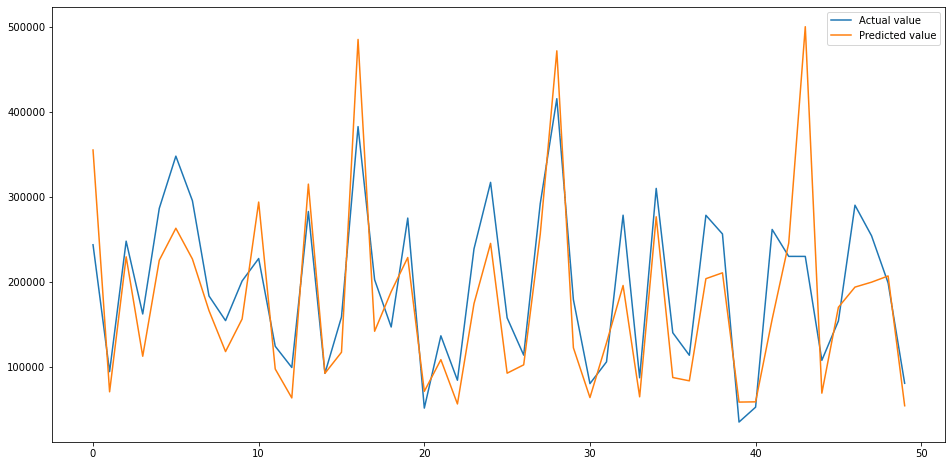That’s the basics of creating a linear regression model in Scikit-Learn. These are the very first steps in creating regression models, but we still got decent results with relatively minimal effort.

Matt Clarke, Saturday, March 06, 2021

Matt Clarke Matt is an Ecommerce and Marketing Director who uses data science to help in his work. Matt has a Master's degree in Internet Retailing (plus two other Master's degrees in different fields) and specialises in the technical side of ecommerce and marketing.